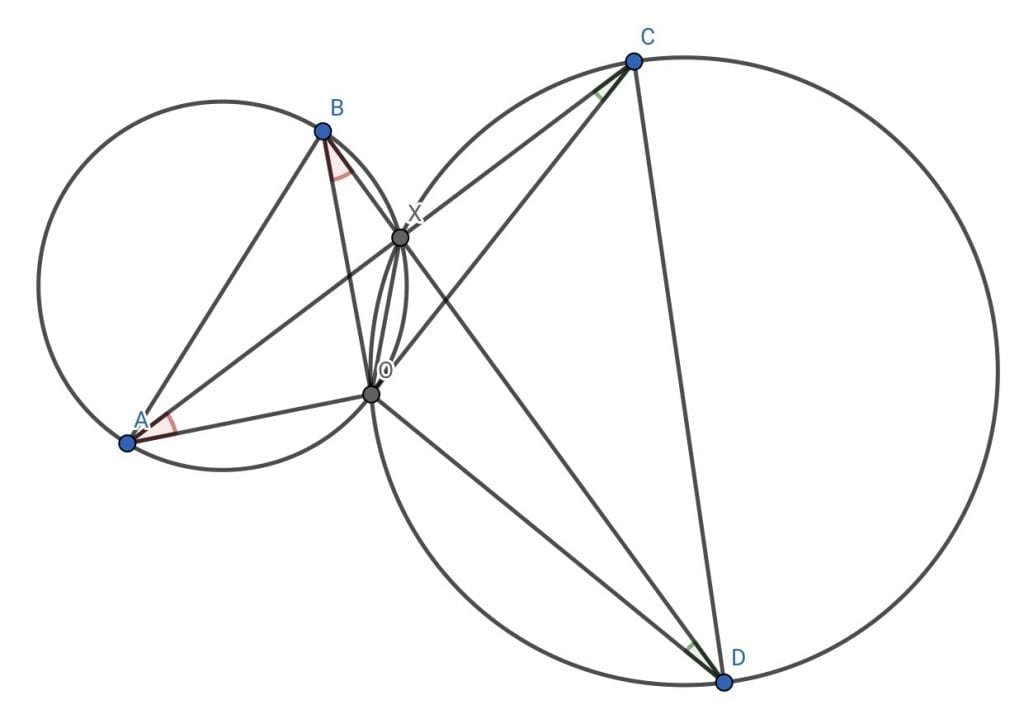How Cheenta works to ensure student success?
Explore the Back-Story

# Spiral Similarity - Complex Number 1# What is spiral similarity?

Spiral similarity, for our purpose, will be a beautiful motion in the plane. It is a combination of rotation and dilation (expansion or contraction by a constant factor.

Suppose AB is a (finite) segment in the plane. CD be another segment. Assume AB and CD to be disjoint.

It is possible to send AB onto CD using a combination of rotation and dilation.To accomplish this, we need to find:

• Center of rotation
• Angle of rotation
• Ratio of dilation

# How to find center of rotation (and dilation)

Join AC and BD. Suppose they intersect at X. Draw the circles CXD and AXB. Let them intersect at O.

O could be same as X or could be a different point.

Claim: O is the center of rotation and dilation, that sends AB to CD.Join OA, OX, OB, OC, OD.

Pause and think: Can you find some cyclic quadrilaterals in this picture.Clearly ABXO is cyclic. This implies $\angle OBX = \angle OAX$. This is because they are subtended on the circumference by the same segment OX. (Angles subtended by a chord at the circumference are equal, by property of cyclic quadrilaterals).

Similarly CDXO is cyclic implying $\angle ODX = \angle OCX$

Pause and think: Can you find a pair of similar triangles?

Hint: Equiangular triangles are similar.

$\Delta OBD \sim \Delta OAC$

This is because we have shown pair of corresponding angles to be equal. Hence the two triangles are equiangular.

Pause and imagine:

This implies $\angle AOC = \angle BOD$. We may imagine this as following:

• OA is turning into OC;
• OB is turning into OD

Since the angles (of rotation) $\angle AOC, \angle BOD$ are equal, hence this works out nicely.

Moreover, OA grows into OC and OB grows into OD by same constant factor. This is true because $\frac {OA}{OC} = \frac{OB}{OD}$. This follows from the similarity of triangles.

Hence A goes to C and B goes to D, via rotation and dilation, centered at O.

# Rotation and dilation is known spiral similarity.

Complex numbers are algebraic tools to encode this motion in the plane.

(This is a supplemental note to live lecture session of Complex Number module of Cheenta I.S.I., C.M.I. Entrance Program and Math Olympiad Program)

# What is spiral similarity?

Spiral similarity, for our purpose, will be a beautiful motion in the plane. It is a combination of rotation and dilation (expansion or contraction by a constant factor.

Suppose AB is a (finite) segment in the plane. CD be another segment. Assume AB and CD to be disjoint.

It is possible to send AB onto CD using a combination of rotation and dilation.To accomplish this, we need to find:

• Center of rotation
• Angle of rotation
• Ratio of dilation

# How to find center of rotation (and dilation)

Join AC and BD. Suppose they intersect at X. Draw the circles CXD and AXB. Let them intersect at O.

O could be same as X or could be a different point.

Claim: O is the center of rotation and dilation, that sends AB to CD.Join OA, OX, OB, OC, OD.

Pause and think: Can you find some cyclic quadrilaterals in this picture.Clearly ABXO is cyclic. This implies $\angle OBX = \angle OAX$. This is because they are subtended on the circumference by the same segment OX. (Angles subtended by a chord at the circumference are equal, by property of cyclic quadrilaterals).

Similarly CDXO is cyclic implying $\angle ODX = \angle OCX$

Pause and think: Can you find a pair of similar triangles?

Hint: Equiangular triangles are similar.

$\Delta OBD \sim \Delta OAC$

This is because we have shown pair of corresponding angles to be equal. Hence the two triangles are equiangular.

Pause and imagine:

This implies $\angle AOC = \angle BOD$. We may imagine this as following:

• OA is turning into OC;
• OB is turning into OD

Since the angles (of rotation) $\angle AOC, \angle BOD$ are equal, hence this works out nicely.

Moreover, OA grows into OC and OB grows into OD by same constant factor. This is true because $\frac {OA}{OC} = \frac{OB}{OD}$. This follows from the similarity of triangles.

Hence A goes to C and B goes to D, via rotation and dilation, centered at O.

# Rotation and dilation is known spiral similarity.

Complex numbers are algebraic tools to encode this motion in the plane.

(This is a supplemental note to live lecture session of Complex Number module of Cheenta I.S.I., C.M.I. Entrance Program and Math Olympiad Program)

This site uses Akismet to reduce spam. Learn how your comment data is processed.

### Knowledge Partner